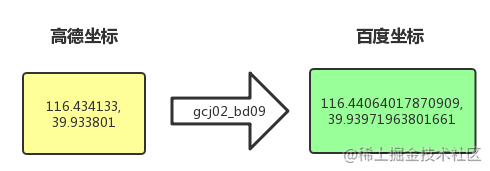# Python 繪制北上廣深的地鐵路線動態圖## 坐標點的采集```url = 'http://map.amap.com/service/subway?_1615466846985&srhdata=1100_drw_beijing.json'
response = requests.get(url)
stations = []
for i in result['l']:
station = []
for a in i['st']:
station.append([float(b) for b in a['sl'].split(',')])
stations.append(station)
pprint.pprint(stations)```

pprint格式化打印結果，方便預覽## 坐標系的轉換```#需要的兩個常量先設置好
pi = 3.1415926535897932384 #π
r_pi = pi * 3000.0/180.0

def gcj02_bd09(lon_gcj02,lat_gcj02):
b = math.sqrt(lon_gcj02 * lon_gcj02 + lat_gcj02 * lat_gcj02) + 0.00002 * math.sin(lat_gcj02 * r_pi)
o = math.atan2(lat_gcj02 , lon_gcj02) + 0.000003 * math.cos(lon_gcj02 * r_pi)
lon_bd09 = b * math.cos(o) + 0.0065
lat_bd09 = b * math.sin(o) + 0.006
return [lon_bd09,lat_bd09]```

```result = []
for station in stations:
result.append([gcj02_bd09(*point) for point in station])```## 地理可視化```from pyecharts.charts import BMap
from pyecharts import options as opts
from pyecharts.globals import BMapType, ChartType ```

```map_b = (
BMap(init_opts = opts.InitOpts(width = "800px", height = "600px"))
baidu_ak = '****************', #百度地圖開發應用appkey
center = [116.403963, 39.915119], #當前視角的中心點
zoom = 10, #當前視角的縮放比例
is_roam = True, #開啟鼠標縮放和平移漫遊
)
series_name = "",
type_ = ChartType.LINES, #設置Geo圖類型
data_pair = result, #數據項
is_polyline = True, #是否是多段線，在畫lines圖情況下#
linestyle_opts = opts.LineStyleOpts(color = "blue", opacity = 0.5, width = 1), # 線樣式配置項
)
maptype_control_opts = opts.BMapTypeControlOpts(type_ = BMapType.MAPTYPE_CONTROL_DROPDOWN), #切換地圖類型的控件
scale_control_opts = opts.BMapScaleControlOpts(), #比例尺控件
overview_map_opts = opts.BMapOverviewMapControlOpts(is_open = True), #添加縮略地圖
)
)

map_b.render(path = 'subway_beijing.html')```## 其他效果展示

### 上海-變色`http://map.amap.com/service/subway?_1615467204533&srhdata=3100_drw_shanghai.json`### 廣州-衛星圖`http://map.amap.com/service/subway?_1615494419554&srhdata=4401_drw_guangzhou.json`### 深圳-個性化配色`http://map.amap.com/service/subway?_1615494473615&srhdata=4403_drw_shenzhen.json`## 小結